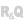HelpWLanguageWLanguage functionsControls, pages and windowsDiagram editor functionsPrefix syntaxTypes of variablesDiagramToImagePresentationExampleSee alsoSaveDiagram constantsDiagram management functionsDiagramEndModificationDiagramGroupDiagramInfoXYDiagramLoadDiagramLoadLibraryDiagramSaveDiagramSearchDiagramSelectMinusDiagramSelectPlusDiagramShapeToImageDiagramStartModificationDiagramToImageDiagramToSVGDiagramUngroup
 WINDEVWEBDEVWINDEV MobileOthersDiagramToImage (Function) In french: DiagrammeVersImage Exports a diagram to a variable of type Image. The generated image can then be saved or displayed in another control.Example // Save a diagram to a PNG fileDiagImage is ImageDiagImage = DiagramToImage(DIAGEDT_MyDiagram.Value)IF ErrorOccurred THEN Error() RETURNENDdSaveImagePNG(DiagImage, "DiagramExport.png") MyDiagram is Diagram <- DIAGEDT_MyDiagram // Retrieves the generated imageMyImage is Image <- DiagramToImage(MyDiagram) // Open the imageOpenDocument(MyImage) Syntax = DiagramToImage( [, ]) : Image variableVariable of type Image corresponding to the image of the diagram. : Name of the control or Diagram variableDiagram to be used. This parameter corresponds to: the name of a Diagram Editor control. the name of a variable of type Diagram. : Optional integerImage background color. This color can correspond to:an RGB color (returned by RGB),an HSL color (returned by HSL),a WLanguage preset color,a variable of type Color. If the background color is not specified, the diagram will be exported as is, with the background color already defined (if any).Component: wd280mdl.dll Minimum version required Version 27 This page is also available for…